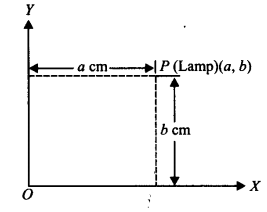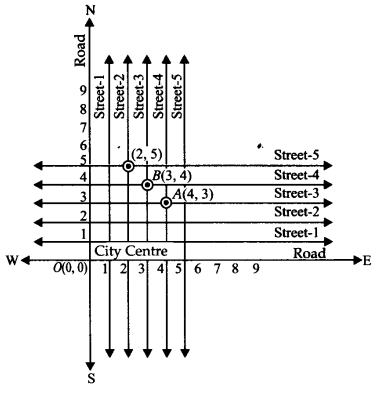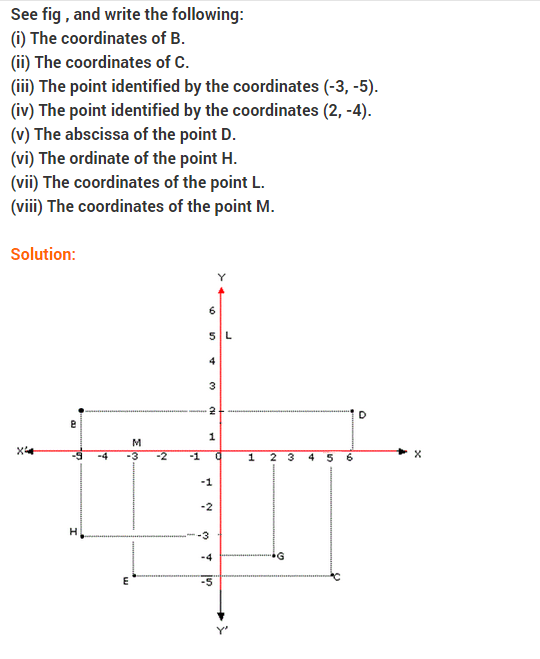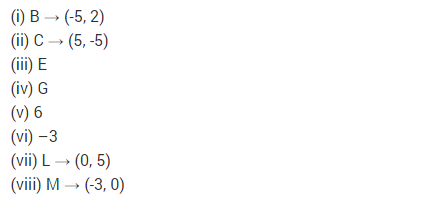# NCERT Solutions for Class 9 Maths Chapter 3 Coordinate Geometry Ex 3.1

NCERT Solutions for Class 9 Maths Chapter 3 Coordinate Geometry Ex 3.1

## NCERT Solutions for Class 9 Maths Chapter 3 Coordinate Geometry Ex 3.1

Ex 3.1 Class 9 Maths Question 1.
How will you describe the position of a table lamp on your study table to another person?
Solution:
To describe the position of a table lamp placed on the table, let us consider the table lamp as P and the table as a plane.
Now choose two perpendicular edges of the table as the axes OX and OY.

Measure the perpendicular distance ‘a’cm of P (lamp) from OY. Measure the perpendicular distance ‘b’ cm of P (lamp) from OX.
Thus, the position of the table lamp P is described by the ordered pair (a, b).Ex 3.1 Class 9 Maths Question 2.
(Street Plan): A city has two main roads which cross each other at the centre of the city. These two roads are along the North-South direction and East-West direction. All other streets of the city run parallel to these roads and are 200 m apart. There are 5 streets in each direction. Using 1 cm = 200 m, draw a model of the city on your notebook. Represent the roads/streets by single lines.
There are many cross-streets in your model. A particular cross-street is made by two streets, one running in the North-South direction and another in the East-West direction. Each cross street is referred to in the following manner: If the 2nd street running in the North-South direction and 5th in the East-West direction meet at some crossing, then we will call this cross-street (2,5). Using this convention, find:
(i) how many cross-streets can be referred to as (4,3).
(ii) how many cross-streets can be referred to as (3,4).
Solution:
(i) A unique cross street as shown by the point A(4, 3).
(ii) A unique cross street as shown by the point B(3,4).
The two cross streets are uniquely found because of the two reference lines we have used for locating them.### NCERT Solutions for Class 9 Maths Chapter 3 Coordinate Geometry Ex 3.2### NCERT Solutions for Class 9 Maths Chapter 3 Coordinate Geometry Ex 3.3## Coordinate Geometry Class 9 Extra Questions Maths Chapter 3

Extra Questions for Class 9 Maths Chapter 3 Coordinate Geometry## SabDekho

The Complete Educational Website